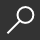## Elements of Algebra by Leonard Euler and John Hewlett

Overview -
In 1770, one of the founders of pure mathematics, Swiss mathematician Leonard Euler (1707-1783), published Elements of Algebra, a mathematics textbook for students. This edition of Euler's classic, published in 1822, is an English translation which includes notes added by Euler's tutor, Johann Bernoulli, and additions by Joseph-Louis Lagrange, both giants in eighteenth-century mathematics, as well as a short biography of Euler. Part 1 begins with elementary mathematics of determinate quantities and includes four sections on simple calculations (adding, subtracting, division, multiplication), and then progresses to compound calculations (fractions), ratios and proportions and algebraic equations. Part 2 consists of 15 chapters on analyses of indeterminate quantities. Here, Euler shows the reader several ways to solve polynomial equations up to the fourth degree. This landmark book showed students the beauty of mathematics, and more significantly, how to do it.

Paperback - Revised Ed.
• \$62.99
local_shippingFor DeliveryIn Stock.
This item is Non-Returnable.
FREE Shipping for Club Members helpNew & Used Marketplace 7 copies from \$63.65

## More About Elements of Algebra by Leonard Euler; John Hewlett

### Overview

In 1770, one of the founders of pure mathematics, Swiss mathematician Leonard Euler (1707-1783), published Elements of Algebra, a mathematics textbook for students. This edition of Euler's classic, published in 1822, is an English translation which includes notes added by Euler's tutor, Johann Bernoulli, and additions by Joseph-Louis Lagrange, both giants in eighteenth-century mathematics, as well as a short biography of Euler. Part 1 begins with elementary mathematics of determinate quantities and includes four sections on simple calculations (adding, subtracting, division, multiplication), and then progresses to compound calculations (fractions), ratios and proportions and algebraic equations. Part 2 consists of 15 chapters on analyses of indeterminate quantities. Here, Euler shows the reader several ways to solve polynomial equations up to the fourth degree. This landmark book showed students the beauty of mathematics, and more significantly, how to do it.

This item is Non-Returnable.

### Details

• ISBN-13: 9781108002967
• ISBN-10: 110800296X
• Publisher: Cambridge University Press
• Publish Date: July 2009
• Page Count: 628
• Dimensions: 8.5 x 5.51 x 1.39 inches
• Shipping Weight: 1.73 pounds

Series: Cambridge Library Collection - MathematicsRelated Categories